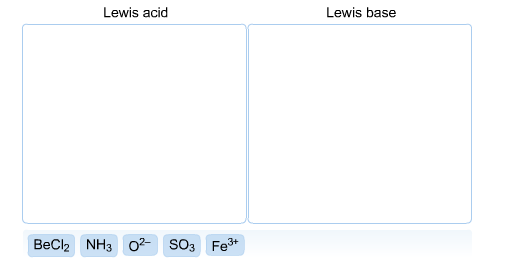# Problem: Classify each of the following as a Lewis acid or a Lewis base.

###### FREE Expert Solution

A Lewis acid is an electron acceptor. All cations are Lewis acids (Ca+2, Fe+3). Atoms or ions that have an incomplete octet can also act as Lewis acids (BF3, AlF3). Molecules whose central atom can hold more than 8 electrons can also be electron acceptors and are classified as Lewis acids (SiF4).

A Lewis base is an electron donor. They tend to be ions that are negatively charged (F-, O2-). Molecules with electrons on their central atom (NH3) can also be Lewis bases.###### Problem Details

Classify each of the following as a Lewis acid or a Lewis base.What scientific concept do you need to know in order to solve this problem?

Our tutors have indicated that to solve this problem you will need to apply the Lewis Acid and Base concept. You can view video lessons to learn Lewis Acid and Base. Or if you need more Lewis Acid and Base practice, you can also practice Lewis Acid and Base practice problems.

What is the difficulty of this problem?

Our tutors rated the difficulty ofClassify each of the following as a Lewis acid or a Lewis ba...as medium difficulty.

How long does this problem take to solve?

Our expert Chemistry tutor, Sabrina took 6 minutes and 23 seconds to solve this problem. You can follow their steps in the video explanation above.

What professor is this problem relevant for?

Based on our data, we think this problem is relevant for Professor Wasinger's class at CSU CHICO.